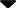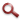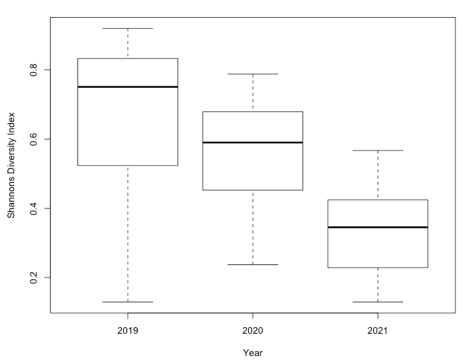Site WebYou are here:

## Student Project FiguresFigure 4 Figure 4: Boxplot depicting the spread of the Shannon's diversity index across the three years. 2019 is clearly the highest (mean = 0.67), followed by 2020 (mean = 0.57), and 2021 has the lowest (mean = 0.35). Significant difference between 2019 and 2021 (p value <0.001), and a significant difference between 2020 and 2020 ( p value = <0.001). There is also a significant difference between 2020 and 2019 (p value = 0.03). Figure 3 Figure 4 Figure 5 Figure 6 Figure 7